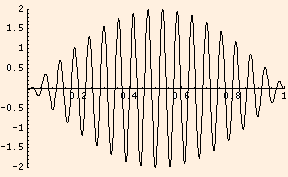### Superposition

1. Using sin a + sin b = 2 cos ((a - b) / 2) sin ((a + b) / 2):
A sin(k x - ω1 t) + A sin(k x - ω2 t + φ)
= 2A cos((ω1 - ω2) t / 2 + φ / 2) sin(k x - (ω1 + ω2) t / 2 + φ / 2)
This solution, with ω2 = - ω1 (a superposition of a left mover and a right mover of equal frequency), is a standing wave:
2A cos (ω1 t + φ / 2) sin (k x + φ / 2)
The value of the
wave number is constrained by the boundary conditions. For a standing wave of length L:

• closed-closed: k = n π / L (n half wavelengths fit in L)
• closed-open: k = n π / (2 L), n odd (n quarter wavelengths fit in L)
• open-open: k = n π / L, φ = π

Think of the second factor as describing a "static standing wave", and the first factor as its time-dependent amplitude.

2. At a fixed position (here with k x = π / 2 and φ = 0), with ω1 independent of ω2, we have beats
2A cos((ω1 - ω2) t / 2 ) cos((ω1 + ω2) t / 2)
with beat frequency ω1 - ω2:Here, the first factor describes the "envelope" and the second describes the oscillation inside; the picture shows one half of an envelope cycle, which is heard as a single beat.

3. This applet will demonstrate beats. This one will demonstrate standing waves (the mode is "n" in the boundary constraints). This applet demonstrates the constructive and destructive interference in traveling waves.
For constructive interference, the phase difference must be an even multiple of π.
For destructive interference, the phase difference must be an odd multiple of π.

 (prev) (top) (next)